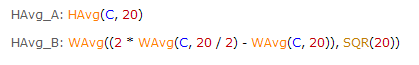Navigation: Realtest Script Language > Formula Syntax

Many elements of a RealTest script allow a formula expression to be provided to calculate the value to be used.

Examples of places where formulas are allowed include:

all the items in the Data, Scan, Results, Graphs, Trades and Charts sections

most of the elements of the Strategy section (see the Strategy Element Table for specifics)

the ExcludeIf element of the Import section

the SkipTestIf and TestName elements of the Settings section

the Debug Panel

the Plot Options dialog

RealTest formula syntax is straightforward and will seem familiar if you’ve used any other testing or scanning software, or ever written an Excel cell formula.

All expressions (including rolling time-series functions) are fully recursive (able to be nested), placing no limits on the type of any argument.

Every term in every function (even bar offset specifications) can be its own formula.

For example, RealTest includes both weighted moving average (WAvg) and Hull moving average (HAvg) built-in functions in addition to the more common simple (MA) and exponential (EMA) flavors.

With the WAvg function and recursive syntax, it was not really necessary to add the built-in HAvg (nevertheless it is there).

The two formulas below will produce the same result:As an alternative to writing deeply nested expressions, you can use the Data section to calculate various parts of a complex rule, and then refer to them by name in other formulas, as shown in many of the Example Scripts.

If a formula cannot be evaluated, the return value will be "nan" (not a number).

The only reasons that a formula cannot be evaluated are:

not enough bars were available to fulfill its lookback plus offset length (unless UseAvailableBars was specified)

it refers to a specific symbol or strategy that is not available in the current data file

Once a formula has returned nan, all other formulas that contain or reference it will also be nan.

If desired, you can use IsNan function to see if a formula would return nan, and/or the NoNan function to force a formula to return 0 instead of nan.

Data Section formulas that return nan will store nan as that data item, so all references to it will be nan.

Scan or Trades Section formulas that return nan will display that item as "nan".

Strategy Element formulas that return nan are treated the same as formulas that return 0, so in general you don't need to worry about nan in your strategy formulas.